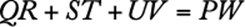﻿ ﻿Geometry Problems - Word Problems - Math Review - GMAT Quantitative Review

## 3.0 Math Review

### 8. Geometry Problems

The following is an example of a word problem involving geometry.

Example:The figure above shows an aerial view of a piece of land. If all angles shown are right angles, what is the perimeter of the piece of land?

Solution: For reference, label the figure asIf all the angles are right angles, then, and. Hence, the perimeter of the land is.

﻿